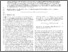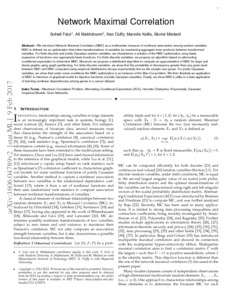# Network Maximal Correlation

Feizi, Soheil and Makhdoumi, Ali and Duffy, Ken R. and Kellis, Manolis and Medard, Muriel (2017) Network Maximal Correlation. IEEE Transactions on Network Science and Engineering, 4 (4). pp. 229-247. ISSN 2327-4697Previewmore...Add this article to your Mendeley library

## Abstract

We introduce Network Maximal Correlation (NMC) as a multivariate measure of nonlinear association among random variables. NMC is defined via an optimization that infers transformations of variables by maximizing aggregate inner products between transformed variables. For finite discrete and jointly Gaussian random variables, we characterize a solution of the NMC optimization using basis expansion of functions over appropriate basis functions. For finite discrete variables, we propose an algorithm based on alternating conditional expectation to determine NMC. Moreover we propose a distributed algorithm to compute an approximation of NMC for large and dense graphs using graph partitioning. For finite discrete variables, we show that the probability of discrepancy greater than any given level between NMC and NMC computed using empirical distributions decays exponentially fast as the sample size grows. For jointly Gaussian variables, we show that under some conditions the NMC optimization is an instance of the Max-Cut problem. We then illustrate an application of NMC in inference of graphical model for bijective functions of jointly Gaussian variables. Finally, we show NMC's utility in a data application of learning nonlinear dependencies among genes in a cancer dataset.

Item Type: Article This is the preprint version (arXiv:1606.04789) of the published article, which is available at DOI: 10.1109/TNSE.2017.2716966 Maximum correlation problem; alternating conditional expectation (ACE); Hermite-Chebyshev polynomials; Gaussian graphical models; gene networks; Faculty of Science and Engineering > Research Institutes > Hamilton Institute 10167 https://doi.org/10.1109/TNSE.2017.2716966 Dr Ken Duffy 01 Nov 2018 17:03 IEEE Transactions on Network Science and Engineering IEEE YesItem control page